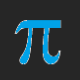## About RobertLovesPi

I go by RobertLovesPi on-line, and am interested in many things, a large portion of which are geometrical. Welcome to my little slice of the Internet. The viewpoints and opinions expressed on this website are my own. They should not be confused with the views of my employer, nor any other organization, nor institution, of any kind.

# Eight Overlapping {8/3} Star Octagons

These octagrams were rotated about the center point. Every other one is yellow, and between the yellow ones are blue ones. The green areas are where the blue and yellow octagrams coincide.

# A Euclidean Construction of a Regular Convex Pentagon and a Regular Star Pentagon, Both Inscribed Inside a Given Circle

1. Start with a (green) circle centered on A with radius AB. Point B is on this circle.
2. Construct a line which intersects line AB at point A, in such a way that these two lines are perpendicular.
3. This green circle intersects the newest line at two points. Designate one of these intersections as point C.
4. Bisect segment AC, and mark point D as this segment’s midpoint.
5. Construct a circle (the blue one) which is centered on D and includes point B.
6. The blue circle intersects line AC at two points. Only one of these points is inside the green circle. Label this point F.
7. Construct segment BF. This segment’s length is the edge length of the pentagon under construction.
8. Construct a circle (the red one) which is centered on B and includes point F.
9. The red and green circles have two points of intersection. One of them is closer to point F than the other; label this closer intersection as point I. The other point of intersection is closer to point C; label it J.
10. J, B, and I are vertices of a regular pentagon. Construct a circle (orange) which is centered on point I and passes through point B. The orange and green circles intersect at two points, one of which is labeled B. Label the other one K. Point K is a vertex of the pentagon under construction.
11. Construct a (purple) circle centered on K and passing through I. This purple circle intersects the green circle at two points, one of which is already labeled I. Label the other point of intersection as L. Point L is the fifth vertex of the pentagon.
12. Connect points with segments to form regular pentagon JBIKL.
13. Connect points with segments to form regular star pentagon BKJIL.

# A 242-Faced Polyhedron, Along With Its Dual

This polyhedron’s faces include twelve decagons, thirty rhombi, and 200 triangles. Its dual is shown below.

This dual has 180 faces: 60 hexagons, and 60 each of two types of kites.

# A Symmetrohedron Featuring Squares, Regular Hexagons, and “Bowtie” Pairs of Trapezoids

This symmetrohedron has eight hexagonal faces, six square faces, and twelve pairs of trapezoids, for a total of 38 faces. I made it by using Stella 4d to modify a truncated octahedron. You may try this program for free at www.software3d.com/Stella.php.